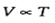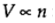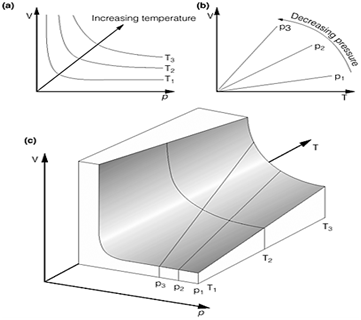## Perfect gases - states of matter, Chemistry

Assignment Help:

A gas is a fluid. It has no resistance to change of shape and will expand indefinitely to fill any container in which is held. The molecules or atoms which make up a gas interact only weakly with each another. They move rapidly, and collide randomly and chaotically with one another. The physical properties of a perfect gas are completely described by four parameters which, with their respective SI units are:

?The amount of substance of which it is comprised, n, in moles;

?The temperature of the gas, T, in Kelvin;

?The pressure of the gas, p, in Pascal;

?The volume occupied by the gas ,V, in m3.

The perfect gas equations

Several separate gas laws were independently developed:

Boyle's law:    p.V=constant               (at constant temperature)

Charles' law:(at constant pressure)

Avogadro's principle:(at constant pressure and temperature)

These three laws are combined in the perfect gas equation of state (also known as the ideal gas law or the perfect gas equation)

The perfect gas equation

pV=nRT

The four parameters of perfect gas are not independent and the relations between parameters are expressed in the gas laws. The perfect gas laws are unified into a single equation of state for a gas which fully expresses the relationships between all four properties. These relationships, however, are based on approximations to experimental observations and only apply to a perfect gas.

Partial pressure:

The pressure of a mixture of gases in a particular volume is the sum of the partial pressure of each individual constituent gas, with the partial pressure of each gas being the pressure that it would exert if it alone occupied the same volume. Dalton's law is strictly true only for ideal gases

The total pressure exerted by a mixture of ideal gases is related to the partial pressures through Dalton's law, which may be illustrating as:

"The total pressure exerted by a mixture of ideal gases in a volume is equal to the arithmetic sum of the partial pressures".Fig.1. Graphical representations of the ideal gas equations. (a) Boyle's law; (b) Charles' law;(c) The surface

assignment

#### 2nd law of thermodynamics, Initially 5 mol of an ideal gas with Cv,m=12.5 J...

Initially 5 mol of an ideal gas with Cv,m=12.5 J K^-1 mol^-1 are at a volume of 5 dm^3 and a temperature of 300K. If the gas is heated to 373 K and the volume changed to 10 dm^3, w

#### Solutions, explain roults law

explain roults law

#### Corrosion, classification corrosion

classification corrosion

#### Solid state, how many solid state are there?

how many solid state are there?

#### #titl, Fe+ AgNO3-->Fe(NO3)2 + Ag

Fe+ AgNO3-->Fe(NO3)2 + Ag

#### #title.boiler corrosion, explain main reason for boiler corrosion ?

explain main reason for boiler corrosion ?

#### Visualisation of hybrid orbitals, Visualisation of hybrid orbitals

Visualisation of hybrid orbitals

what is an acid

#### Bond theory, describe valence bond theory and its limitations

describe valence bond theory and its limitations